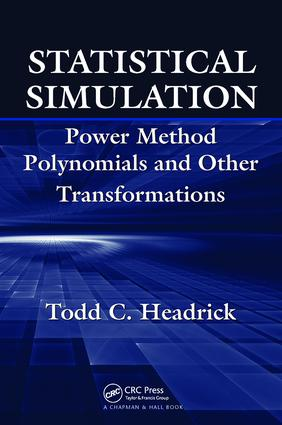# Statistical Simulation

## Power Method Polynomials and Other Transformations, 1st Edition

Chapman and Hall/CRC

174 pages | 6 B/W Illus.

##### Purchasing Options:\$ = USD
Paperback: 9781138116283
pub: 2017-06-14
\$82.95
x
Hardback: 9781420064902
pub: 2009-12-08
Currently out of stock
\$220.00
x
eBook (VitalSource) : 9780429144370
pub: 2009-12-08
from \$39.98

FREE Standard Shipping!

### Description

Although power method polynomials based on the standard normal distributions have been used in many different contexts for the past 30 years, it was not until recently that the probability density function (pdf) and cumulative distribution function (cdf) were derived and made available. Focusing on both univariate and multivariate nonnormal data generation, Statistical Simulation: Power Method Polynomials and Other Transformations presents techniques for conducting a Monte Carlo simulation study. It shows how to use power method polynomials for simulating univariate and multivariate nonnormal distributions with specified cumulants and correlation matrices.

The book first explores the methodology underlying the power method, before demonstrating this method through examples of standard normal, logistic, and uniform power method pdfs. It also discusses methods for improving the performance of a simulation based on power method polynomials. The book then develops simulation procedures for systems of linear statistical models, intraclass correlation coefficients, and correlated continuous variates and ranks. Numerical examples and results from Monte Carlo simulations illustrate these procedures. The final chapter describes how the g-and-h and generalized lambda distribution (GLD) transformations are special applications of the more general multivariate nonnormal data generation approach. Throughout the text, the author employs Mathematica® in a range of procedures and offers the source code for download online.

Written by a longtime researcher of the power method, this book explains how to simulate nonnormal distributions via easy-to-use power method polynomials. By using the methodology and techniques developed in the text, readers can evaluate different transformations in terms of comparing percentiles, measures of central tendency, goodness-of-fit tests, and more.

### Reviews

Headrick’s book is a valuable addition to the simulation world in the sense that it is the first systematic book on power method polynomials … this book has potential to be one of the key sources for researchers who are involved in random number generation and Monte Carlo studies. … it can serve and seems to be designed as a supplemental text in graduate level simulation- and computation-oriented courses. … Mathematica functions are provided throughout the book, which is a nice feature … Headrick’s book is a fairly major contribution to the literature … . I would highly recommend this book to anyone who deals with random number generation and more generally with Monte Carlo simulation and statistical computing.

Journal of Statistical Software, Vol. 43, September 2011

This interesting monograph concerns the use of power method polynomials in the context of simulating univariate and multivariate nonnormal distributions with given cumulant and correlation structure. Its strength is in the fact that the power method, g-and-h transformations and GLD transformations are capable of simulation multivariate nonnormal continuous distributions specified by its cumulants and correlation matrices. … —Zentralblatt MATH 1187

Introduction

The Power Method Transformation

Univariate Theory

Third-Order Systems

Fifth-Order Systems

Mathematica® Functions

Limitations

Multivariate Theory

Using the Power Method Transformation

Introduction

Examples of Third- and Fifth-Order Polynomials

Remediation Techniques

Monte Carlo Simulation

Some Further Considerations

Simulating More Elaborate Correlation Structures

Introduction

Simulating Systems of Linear Statistical Models

Methodology

Numerical Example and Monte Carlo Simulation

Simulating Intraclass Correlation Coefficients

Methodology

Numerical Example and Monte Carlo Simulation

Simulating Correlated Continuous Variates and Ranks

Methodology

Numerical Example and Monte Carlo Simulation

Other Transformations: The g-and-h and GLD Families of Distributions

Introduction

The g-and-h Family

The Generalized Lambda Distributions (GLDs)

Numerical Examples

Multivariate Data Generation

References

Index

### About the Author

Todd C. Headrick is an associate professor and coordinator of the Educational Statistics & Measurement program at Southern Illinois University Carbondale.

### Subject Categories

##### BISAC Subject Codes/Headings:
MAT029000
MATHEMATICS / Probability & Statistics / General
MAT029010
MATHEMATICS / Probability & Statistics / Bayesian Analysis